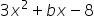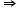Maths-
General
Easy

Question

# What are all the possible values of b for whichis it factorable using only integer coefficients and constant?Hint:

## The correct answer is: {-23,-10,-5,-2,2,5,10,23} are all possible values of b.

### Ans:-  {-23,-10,-5,-2,2,5,10,23} are all possible values of b.Given,is it factorable  so be must be sum of factors of (-8)(3)Factors of -24 are(1,-24)b can be -24 + 1 = -23(2,-12)b can be -12 + 2 = -10(3,-8)b can be -8 + 3 = -5(4,-6)b can be 4 - 6 = -2(6,-4)b can be 6 - 4 = 2(8,-3)b can be 8 - 3 = 5(12,-2)b can be 12 - 2 = 10(24,-1)b can be 24 - 1 = 23So, there are 8 possible values of b they are {-23, -10, -5, -2, 2, 5, 10, 23}#### With Turito Foundation.#### Get an Expert Advice From Turito.